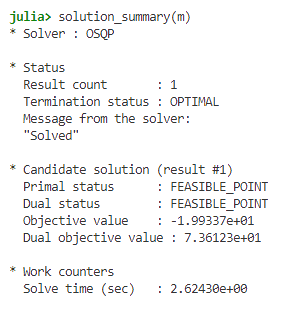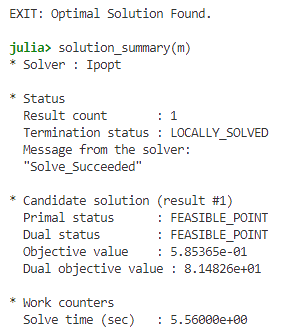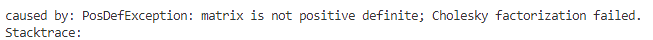# Getting HiGHs to return when objective value doesn't move

I’m trying to solve a constrained optimization problem with JuMP and HiGHs (this is for an open source package so I want to rely on an open source solver), but for some reason the optimization never terminates although the objective value reaches a minimum very quickly.

I’m posting an MWE (thanks to the invaluable help of @jd-foster on Slack) with real world data below. The problem to solve is:

\begin{align} \min_{w} \left| \left| Z - \sum_{j} w_jX_j \right| \right|^2 + \lambda \sum_{j}w_j \left| \left| Z - X_j \right| \right|^2 \\ s.t. \ 0 \leq w_j \leq 1 \ \forall \ j \\ \sum_{j} w_j = 1 \end{align}

where Z is a length-k vector, and X is a (k \times J) matrix. The idea is that Z is an observation with k characteristics, and X holds J other observations with measurements on the same characteristics. The objective is to find a weighted combination of observations (columns) in X to approximate Z, with the first term in the objective penalising the overall distance between Z and the weighted average and the second term the pair-wise distance between Z and the columns of X included in the weighted average.

(For those interested this is the “Penalized Synthetic Control” estimator from Abadie and L’Hour (2021))

The runnable code is:

using Downloads, DelimitedFiles, LinearAlgebra
import JuMP: JuMP, Model, @variable, @constraint, @objective
import HiGHS

Z = [5.17119501, 5.4708669, 2.5463325, 2.7430484, 0.0, 0.0, 0.0, 2.19362429, 2.19362429, 2.03571710]

function create_model(Z, X, λ; optimizer=HiGHS.Optimizer)

model = Model(optimizer)
J = size(X, 2)
@variable(model, 0 <= w[1:J] <= 1)
@constraint(model, sum(w[i] for i ∈ 1:J) == 1)
@objective(model, Min,
sum( (Z .- X*w).^2 ) + λ * sum( w[j]* sum( (Z .- X[:,j])).^2 for j in 1:J)
)

return model
end

m = create_model(Z, X, 0.1)
JuMP.set_attribute(m, "time_limit", 120.0)
JuMP.optimize!(m)


which for me gives:

Iteration, Runtime, ObjVal, NullspaceDim
0, 0.356691, 24.143424, 0
1000, 86.010277, 0.587094, 7
1386, 120.059697, 0.587094, 6
Model   status      : Time limit reached
QP ASM    iterations: 1386
Objective value     :  5.8709427083e-01
HiGHS run time      :        120.09


Playing around with the time_limit I found that the final objective value is actually reached after ~20 iterations or around 2 seconds, and the solver keeps running forever afterwards (I’ve let it run for about an hour before terminating).

It seems to me that there should be a way to get HiGHs to return here, but playing around with the various gap or tolerance related parameters doesn’t seem to do anything.

Lastly, @jd-foster solved this problem in Gurobi which suggested perturbing the diagonal term in the quadratic objective. This is not something I’d like to do in my package as default (as the diagonal is meaningful and users are likely to feed in Z and X in standardised in such a way that they expect an identity diagonal) but it might help diagnose the issue that HiGHs is facing here.

In summary, my questions are:

1. How can I get HiGHs to return a value when the objective stops changing; and
2. Are there any strategies to make my solution strategy more robust to whatever is happening here in the context of a package which should be able to deal with all sorts of user provided data?
3 Likes

I changed the solver to OSQP (also open source, but not for mixed integer problems - in case that matters) and I got a solution in a few seconds:The value of the objective is different in OSQP and in HiGHS, though. When I run the code with HiGHS, I get similar results to you. However, when I check the solution summary, I see that the duals are infeasible:

So possibly a reason for not finishing is this infeasibility. I don’t know if the infeasibility is a bug in HiGHS or a property of the problem. Just for the sake of completeness, Ipopt was able to find the same solution as HiGHS:with similar dual values.

And SCS failed complaining that the matrix is not positive definite:I am not sure a linear solver or a solver requiring positive semi-definite matrix Q can be robust to all sorts of user provided data. Maybe the way to go is to check whether the matrices created from data satisfy the assumptions of a solver and warn the user if this is not the case?

There are a couple of things going on here.

The matrix has a large condition number:

λ = 0.1
model = Model()
J = size(X, 2)
@variable(model, 0 <= w[1:J] <= 1);
expr = JuMP.@expression(model,
sum( (Z .- X*w).^2 ) + λ * sum( w[j]* sum( (Z .- X[:,j])).^2 for j in 1:J)
);
vmap = Dict(w[j] => j for j in 1:J);
Q = zeros(J, J);
for (k, v) in expr.terms
Q[vmap[k.a], vmap[k.b]] += v
Q[vmap[k.b], vmap[k.a]] += v
end

julia> LinearAlgebra.cond(Q)
4.079491602024465e22


The QP solver in HiGHS is immature and still seeing development/bug fixes.

I’d use Gurobi or Ipopt, and I’d write your model like this:

function create_model2(Z, X, λ; optimizer=Ipopt.Optimizer)
model = Model(optimizer)
J = size(X, 2)
@variable(model, 0 <= w[1:J] <= 1)
@constraint(model, sum(w) == 1)
@variable(model, residuals[1:length(Z)])
@constraint(model, residuals .== Z .- X*w)
coefficients = [sum(Z .- X[:,j]).^2 for j in 1:J]
@objective(model, Min, sum(residuals.^2) + λ * coefficients' * w)
return model
end


This moves the data into linear equality constraints (easy) and it simplifies the quadratic to a diagonal matrix.

2 Likes

This works very well from my testing; it has the equivalent objective and solution as the other versions, and is a few orders of magnitude quicker as bonus.

MWE for anyone interested:

import DelimitedFiles
import JuMP: JuMP, Model, @variable, @constraint, @objective
import Ipopt

Z = [5.1711950141609835, 5.470866932205469, 2.546332563474285, 2.743048443920391, 0.0, 0.0, 0.0, 2.1936242978411693, 2.1936242978411697, 2.0357171080835728]

function create_model2(Z, X, λ; optimizer=Ipopt.Optimizer)
model = Model(optimizer)
J = size(X, 2)
@variable(model, 0 <= w[1:J] <= 1)
@constraint(model, sum(w) == 1)
@variable(model, residuals[1:length(Z)])
@constraint(model, residuals .== Z .- X*w)
coefficients = [sum(Z .- X[:,j]).^2 for j in 1:J]
@objective(model, Min, sum(residuals.^2) + λ * coefficients' * w)
return model
end

function run(Z, X, λ; optimizer=Ipopt.Optimizer, filter_tol = 1e-4)
model2 = create_model2(vec(Z), X, 0.1; optimizer=optimizer)
JuMP.optimize!(model2)

obj_val = JuMP.objective_value(model2)
## Non-trivial values:
sol_nontrivial = [(i, val) for (i, val) in enumerate(JuMP.value.(model2[:w])) if val > filter_tol]

return obj_val, sol_nontrivial
end

@time obj_val, sol = run(Z, X, 0.1);
## 0.294944 seconds (50.57 k allocations: 9.058 MiB)


with

julia> obj_val
0.5853730907635923

julia> sol
7-element Vector{Tuple{Int64, Float64}}:
(13, 0.18656265907917816)
(44, 0.032810328329308656)
(1657, 0.007132053670042325)
(1671, 0.6827712072110597)
(1690, 0.01430815837450065)
(1700, 0.014476627030865307)
(1757, 0.06191521912803008)

1 Like